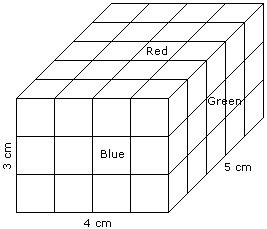# Verbal Reasoning - Cube and Cuboid - Discussion

Discussion Forum : Cube and Cuboid - Cube and Cuboid 3 (Q.No. 3)
Directions to Solve

The following questions are based on the information given below:

1. A cuboid shaped wooden block has 4 cm length, 3 cm breadth and 5 cm height.
2. Two sides measuring 5 cm x 4 cm are coloured in red.
3. Two faces measuring 4 cm x 3 cm are coloured in blue.
4. Two faces measuring 5 cm x 3 cm are coloured in green.
5. Now the block is divided into small cubes of side 1 cm each.

3.
How many small cubes will have no faces coloured ?
None
2
4
6
Explanation:Required number of small cubes = (5 - 2) x (4 - 2) x (3 - 2)

= 3 x 2 x 1

= 6

Discussion:
6 comments Page 1 of 1.

Lokii said:   2 years ago
@All.

I think it should be 12 then because inside we have uncolored 6x2 cubes.

Correct me, if I am wrong.

Kannan said:   3 years ago
Then the formula for cube no face painted is? Please, anyone, explain.

Shofi said:   8 years ago
Its very easy.

No face colour formula (x-2)^3 this formula use only same cube value. But now we have L = 4, b = 3, c = 5.

So we have use (L-2)*(b-2)*(c-2).

Then apply the value (4-2)*(3-2)*(5-2) = 2*1*3 = 6.

Priya said:   8 years ago